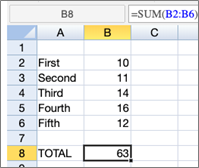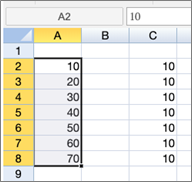enquiries@cenitcollege.ie
01 901 2014

# Time Saving Exam Tip – Use The Spreadsheet Tool

During an exam, making the best use of your time is extremely important. You have put in the time to learn the materials and calculations. But there is one piece of advice that ACCA and CPA examiners constantly point out. The spreadsheet tool is not being used!

Maybe you are not used to working with spreadsheets or worry that the maths will not calculate properly. If the exam you are preparing for allows use of the spreadsheet tool, it may be a good idea to have Microsoft Excel or Google sheets open as you are working through problems. If you are used to organising and solving problems using this tool, you will be a pro during the exam! This blog will walk through some helpful tips and techniques you can use.

The spreadsheet tool looks very similar to Microsoft Excel. By entering and labeling information for the calculations, you can keep your thoughts organised and also show how you arrived at the various parts of your answer. Examiners has expressed that they will often give partial credit for an incorrect answer if they can follow the work and see where an error occurred. If a final answer is simply typed in, they are not able to see this information.

This is an example of how the ACCA questions are organised:

In the ACCA format, you are able to see the various part of the question and resize the windows. For CPA exams, there is typically a tab for “Text Editor” and next to it is the “Spreadsheet” tab. The spreadsheets allow for different formatting options (e.g. bold, italic), as well as the area to input formulas and link to the cells. It is a good idea to become familiar with the percentage and decimal point button tools before the exam, but this blog focuses on equations.

### Step 1

Enter the relevant information from the question into the spreadsheet. For instance, “Total number of units made and sold – 1,400” could be shortened and entered as “Total Units” in one cell, with 1400 entered into the cell next to it. The number must be in a cell by itself so when writing out a formula just the number itself is used.

### Step 2

When you arrive to a spot where a calculation must be done, label first what it will calculate (this is both for you and the examiner). Start by putting an = sign in the cell to begin any equation.

### Step 3

Time to enter the information for the software to calculate! Instead of typing out actual numbers, have the formula pull information from the cells when possible. This avoids errors and saves time. Here are helpful tips and examples:

• To add two cells together, press =, click first cell, +, click second cell, press “enter”
• If you need to add multiple cells from a column of rows, enter =SUM(, (including the first parathesis to start the equation) and click and drag down to include the cells that should be included, then press “enter”:• To subtract, press =, click one cell, -, press another cell
• To divide, use /
• To multiply, use *
• If there is a simple pattern in the numbers (e.g. 10, 20, 30) that should be continued, you can usually highlight the cells, then click and drag the bottom right corner box. This will continue the pattern automatically. This can also be used if you need the same number to repeat.• You can often copy and paste formulas that are linked to cells and re-use the formula. For instance, if a formula in column B links to cell B2, and once copied to the corresponding spot in column C the link would be to cell C2.

** Note that these tips and tricks are based on the functionalities of specimen exam spreadsheets. We assume that they will be available in the same capacity on the actual exam.**

As always, when going through a question try to think through logically to make sure your answers make sense. The spreadsheet tool is a brilliant time saving tool, especially when used appropriately. So, practice as you go through questions while studying. Organise your information and use the formula functions so you will be prepared on the big day!